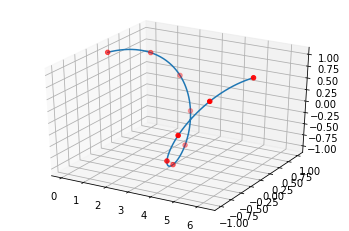4

# Expect x to not have duplicates

Package:
scipy8546
Exception Class:
ValueError

## Raise code

``````            t = _augknt(x, k)

t = _as_float_array(t, check_finite)

if x.ndim != 1 or np.any(x[1:] < x[:-1]):
raise ValueError("Expect x to be a 1-D sorted array_like.")
if np.any(x[1:] == x[:-1]):
raise ValueError("Expect x to not have duplicates")
if k < 0:
raise ValueError("Expect non-negative k.")
if t.ndim != 1 or np.any(t[1:] < t[:-1]):
raise ValueError("Expect t to be a 1-D sorted array_like.")
if x.size != y.shape:
raise ValueError('Shapes of x {} and y {} are incompatible'
.format(x.shape, y.shape))``````
😲 Agile task management is now easier than calling a taxi. #Tracklify
🙏 Scream for help to Ukraine
Today, 2nd July 2022, Russia continues bombing and firing Ukraine. Don't trust Russia, they are bombing us and brazenly lying in same time they are not doing this 😠, civilians and children are dying too! We are screaming and asking exactly you to help us, we want to survive, our families, children, older ones.
Please spread the information, and ask your governemnt to stop Russia by any means. We promise to work extrahard after survival to make the world safer place for all.

## Ways to fix0When using `scipy.interpolate.make_interp_spline` to compute the (coefficients of) interpolating B-spline the x array shouldn't have duplicated value.

## Reproducing the error:

• Install the necessary library

Here numpy and matplotlib are optional. They are used just to show a typical use case of the library and to generate sample data.

```pipenv install scipy numpy matplotlib
```

```from scipy.interpolate import BSpline, make_interp_spline
import numpy as np
from matplotlib import pyplot as plt
ax = plt.axes(projection='3d')
xx = np.linspace(0, 2*np.pi, 100)
x = np.linspace(0, 2*np.pi, 10)
x=0 # here we are making the 10th element the same as the first element, hence creatig redundancy
y = np.array([np.sin(x), np.cos(x)])
bspl = make_interp_spline(x, y, k=5,axis=1)
ax.plot3D(xx, *bspl(xx))
ax.scatter3D(x, *y, color='red')
plt.show()
```

```---------------------------------------------------------------------------
ValueError Traceback (most recent call last)
<ipython-input-10-0386986ac086> in <module>()  7 x=0  8 y = np.array([np.sin(x), np.cos(x)]) ----> 9 bspl = make_interp_spline(x, y, k=5,axis=1)  10 ax.plot3D(xx, *bspl(xx))  11 ax.scatter3D(x, *y, color='red')
/usr/local/lib/python3.7/dist-packages/scipy/interpolate/_bsplines.py in make_interp_spline(x, y, k, t, bc_type, axis, check_finite)  797   798 if x.ndim != 1 or np.any(x[1:] <= x[:-1]): --> 799 raise ValueError("Expect x to be a 1-D sorted array_like.")  800 if k < 0:  801 raise ValueError("Expect non-negative k.")
ValueError: Expect x to be a 1-D sorted array_like.
```

## Fixed:

Avoid the redundant value.

```from scipy.interpolate import BSpline, make_interp_spline
import numpy as np
from matplotlib import pyplot as plt
ax = plt.axes(projection='3d')
xx = np.linspace(0, 2*np.pi, 100)
x = np.linspace(0, 2*np.pi, 10)
# x=0 avoid the redundancy
y = np.array([np.sin(x), np.cos(x)])
bspl = make_interp_spline(x, y, k=5,axis=1)
ax.plot3D(xx, *bspl(xx))
ax.scatter3D(x, *y, color='red')
plt.show()
print(x)
```

## Output: